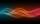# Given

Given 2x =0.125 find the value of x

Result

x =  0.063

#### Solution:

2x=0.125

2x = 0.125

x = 116 = 0.0625

Calculated by our simple equation calculator.

Leave us a comment of example and its solution (i.e. if it is still somewhat unclear...):

Showing 0 comments:Be the first to comment!#### To solve this example are needed these knowledge from mathematics:

Do you have a linear equation or system of equations and looking for its solution? Or do you have quadratic equation?

## Next similar examples:

1. Find xSolve: if 2(x-1)=14, then x= (solve an equation with one unknown)
2. Negative in equation2x + 3 + 7x = – 24, what is the value of x?
3. Boys and girlsThere are 28 girls in the hall. 5/7 of all children are boys. How many children and how many boys are there?
4. Dropped sheetsThree consecutive sheets dropped from the book. The sum of the numbers on the pages of the dropped sheets is 273. What number has the last page of the dropped sheets?
5. Simple equationSolve for x: 3(x + 2) = x - 18
6. Equation 29Solve next equation: 2 ( 2x + 3 ) = 8 ( 1 - x) -5 ( x -2 )
7. Unknown number 11That number increased by three equals three times itself?
8. NormThree workers planted 3555 seedlings of tomatoes in one dey. First worked at the standard norm, the second planted 120 seedlings more and the third 135 seedlings more than the first worker. How many seedlings were standard norm?
9. PearsThere were pears in the basket, I took two-fifths of them, and left six in the basket. How many pears did I take?
10. Simple equationSolve the following simple equation: 2. (4x + 3) = 2-5. (1-x)
11. EquationSolve the equation: 1/2-2/8 = 1/10; Write the result as a decimal number.
12. EqnSolve equation with fractions: 2x/3-50=40+x/4
13. Mysterious numberThe magician thinks the number: "The mysterious number is first divided by minus five, dividing the result by three, multiplying the number by ten, and dividing the resulting number by minus four. This gives result 5. Can you reveal the mysterious number?
14. Unknown numberIdentify unknown number which 1/5 is 40 greater than one tenth of that number.
15. Simplify 2Simplify expression: 5ab-7+3ba-9
16. AlleyAlley measured a meters. At the beginning and end are planted poplar. How many we must plant poplars to get the distance between the poplars 15 meters?
17. HotelThe hotel has a p floors each floor has i rooms from which the third are single and the others are double. Represents the number of beds in hotel.